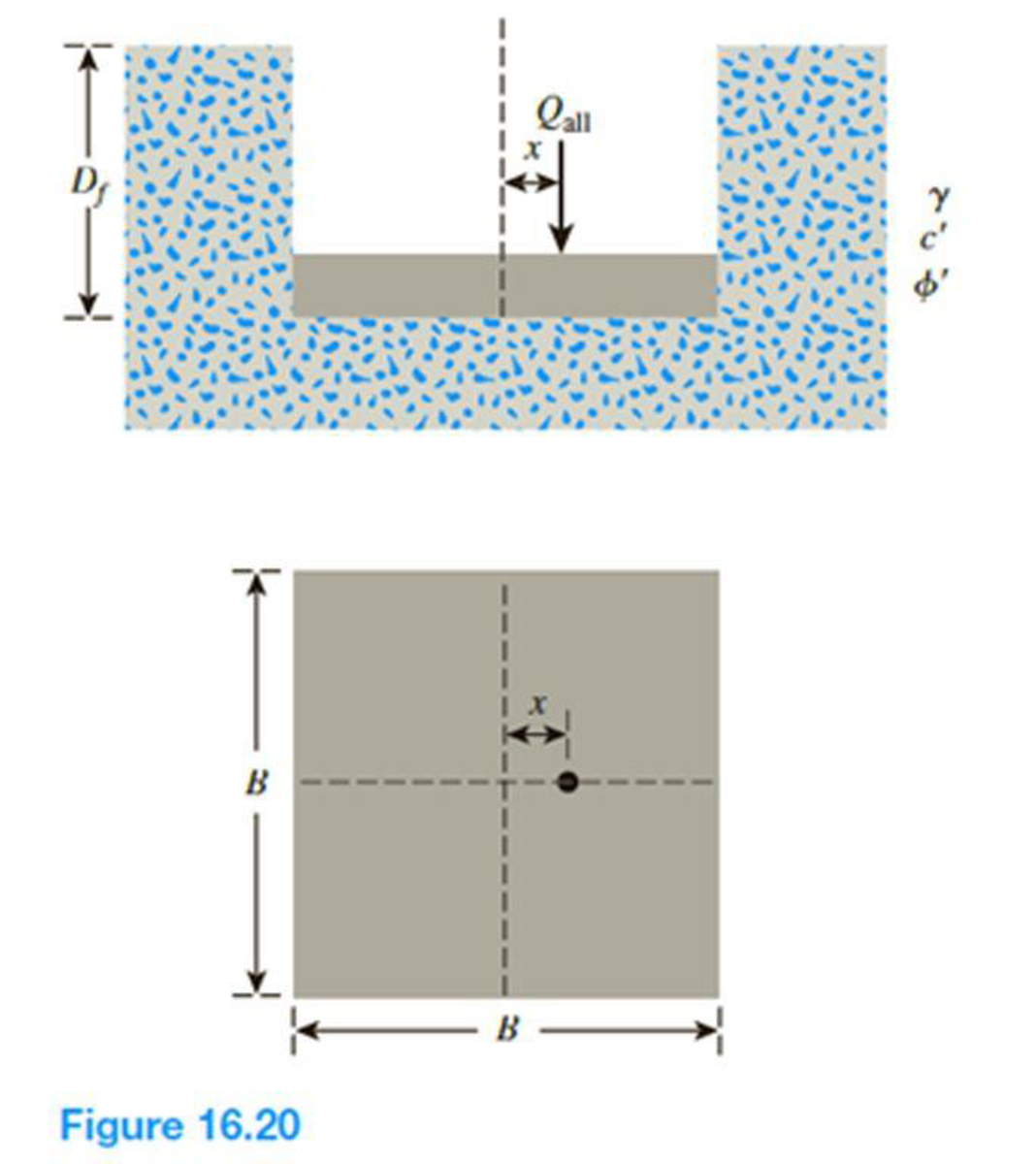Chapter 16, Problem 16.18PPrinciples of Geotechnical Enginee...

9th Edition
Braja M. Das + 1 other
ISBN: 9781305970939

Solutions

Chapter
SectionPrinciples of Geotechnical Enginee...

9th Edition
Braja M. Das + 1 other
ISBN: 9781305970939
Textbook Problem

Refer to the footing in Problem 16.16. Determine the gross ultimate load the footing can carry using the Patra et al. (2015) reduction factor method for rectangular foundations given in Eqs. (16.47), (16.49), and (16.50).16.16 A square footing on sand is subjected to an eccentric load as shown in Figure 16.20. Using Meyerhof’s effective area concept, determine the gross allowable load that the footing could carry with Fs = 4. Given: γ = 16 kN/m3, c′ = 0, ϕ′ = 29°, Df = 1.3 m, B = 1.75 m, and x = 0.25 m. Use Eqs. (16.32) through (16.42) for shape, depth, and inclination factors.To determine

Find the gross ultimate load that the footing can carry using the reduction factor method.

Explanation

Given information:

The unit weight of the soil γ is 16kN/m3.

The value of cohesion c is 0.

The soil friction angle ϕ is 29°.

The location of depth of footing base Df is 1.3 m.

The width of the footing B is 1.75 m.

The value of eccentricity e is 0.25 m.

The factor of safety value Fs is 4.

Calculation:

Determine the reduction factor Rk using the relation.

Rk=a(eB)k (1)

Here, a and k are the functions of the embedment ratio DfB.

Determine the function a using the relation.

a=(BL)21.6(BL)+2.13

Substitute 1.75 m for B and 1.75 m for L.

a=(1.751.75)21.6(1.751.75)+2.13=1.53

Determine the function k using the relation.

k=0.3(BL)20.56(BL)+0.9

Substitute 1.75 m for B and 1.75 m for L.

k=0.3(1.751.75)20.56(1.751.75)+0.9=0.64

Substitute 1.53 for a, 0.25 m for e, 1.75 m for B, and 0.64 for k in Equation (1).

Rk=1.53(0.251.75)0.64=0.44

Determine the shape factor λcs using the relation.

λcs=1+(BL)(NqNc)

Here, Nq and Nc are the bearing-capacity factors.

Refer Table 16.2, “Bearing-capacity factors Nc, Nq, and Nγ” in the textbook.

For ϕ=29°;

The values of Nc is 27.86, Nq is 16.44, and Nγ is 19.34.

Substitute 1.75 m for B, 1.75 m for L, 16.44 for Nq, and 27.86 for Nc.

λcs=1+(1.751.75)(16.4427.86)=1.421

Determine the depth factor λcd using the relation.

λcd=1+0.4tan1(DfB)

Substitute 1.3 m for Df and 1.75 m for B.

λcd=1+0.4(1.31.75)=1.297

Determine the shape factor λqs using the relation.

λqs=1+(BL)tanϕ

Substitute 1.75 m for B, 1.75 m for L, and 29° for ϕ.

λqs=1+(1.751.75)tan29°=1.554

Determine the depth factor λqd using the relation.

λqd=1+2tanϕ(1sinϕ)2(DfB)

Substitute 29° for ϕ, 1

Still sussing out bartleby?

Check out a sample textbook solution.

See a sample solution

The Solution to Your Study Problems

Bartleby provides explanations to thousands of textbook problems written by our experts, many with advanced degrees!

Get Started

How much natural gas do you need to burn to heat twenty gallons of water?

Engineering Fundamentals: An Introduction to Engineering (MindTap Course List)

Briefly describe a ball screw and a linear guide.

Precision Machining Technology (MindTap Course List)

What is a business rule, and what is its purpose in data modeling?

Database Systems: Design, Implementation, & Management

Which security protocols are used to protect e-mail?

Principles of Information Security (MindTap Course List)

Which of the systems are equivalent to the couple in (a)?

International Edition---engineering Mechanics: Statics, 4th Edition

Explain Appleâ€™s view of user interface design, especially for apps.

Systems Analysis and Design (Shelly Cashman Series) (MindTap Course List)

For what other reasons might you share your calendar?

Enhanced Discovering Computers 2017 (Shelly Cashman Series) (MindTap Course List)

Which Transport layer protocol does PPTP use?

Network+ Guide to Networks (MindTap Course List)